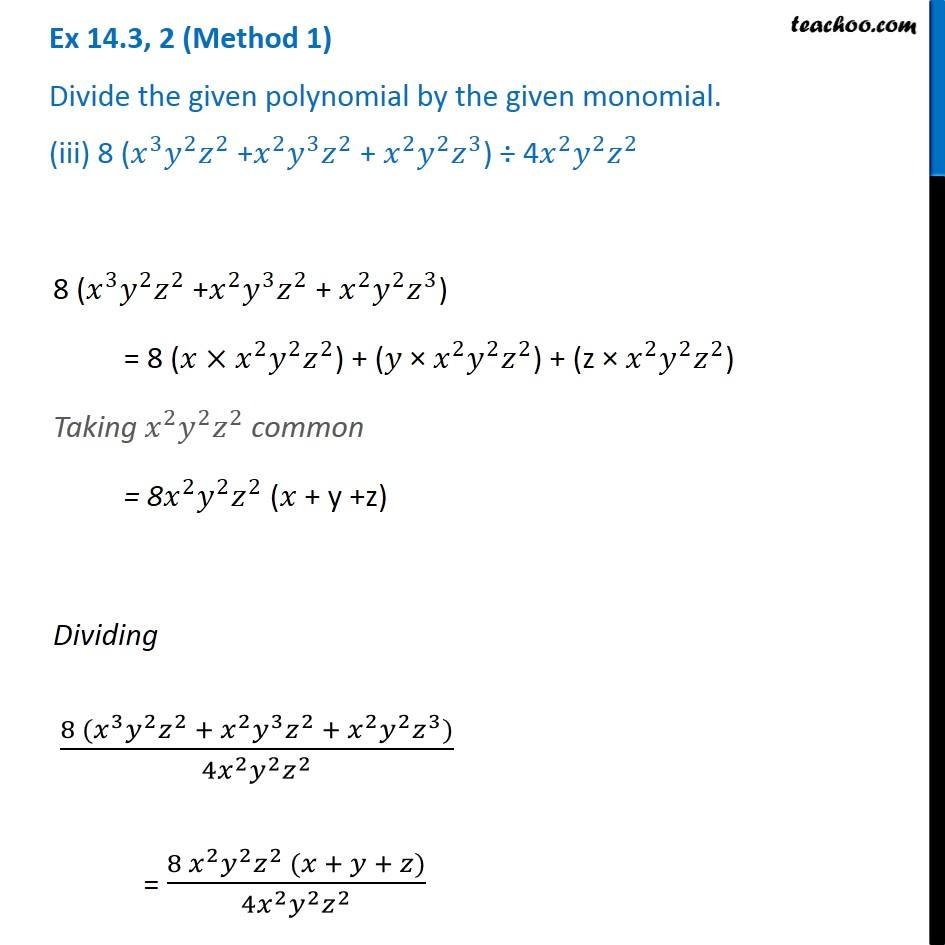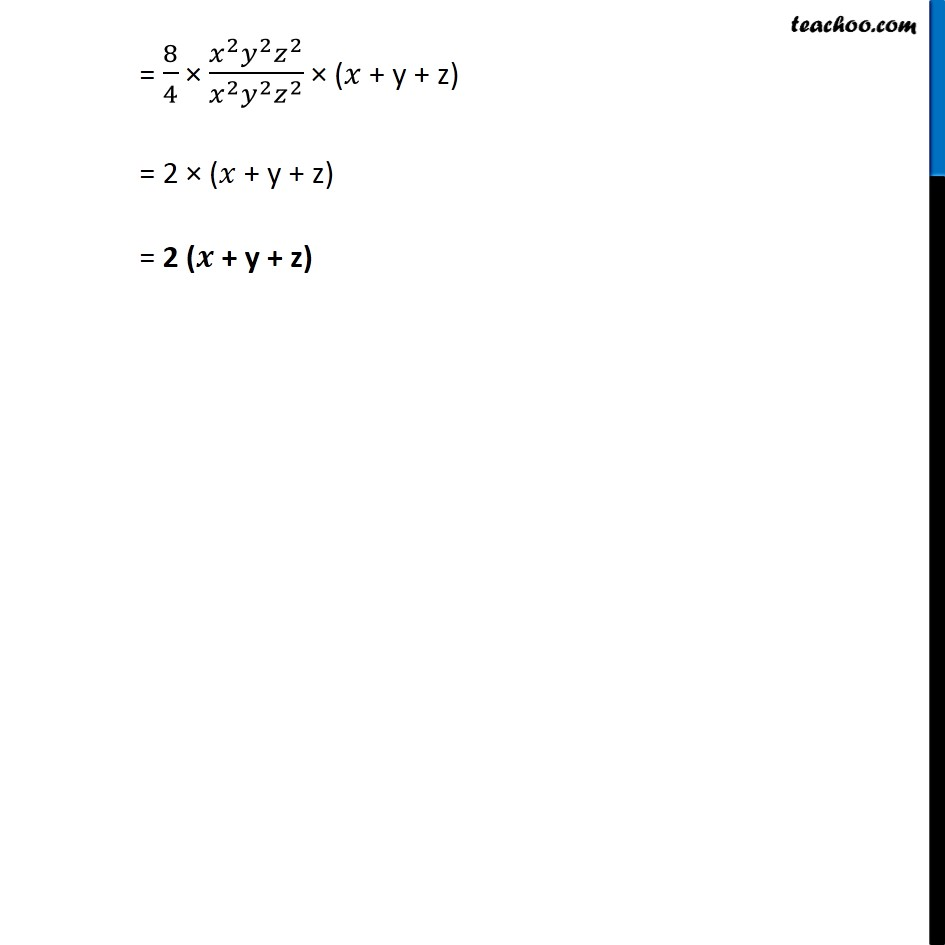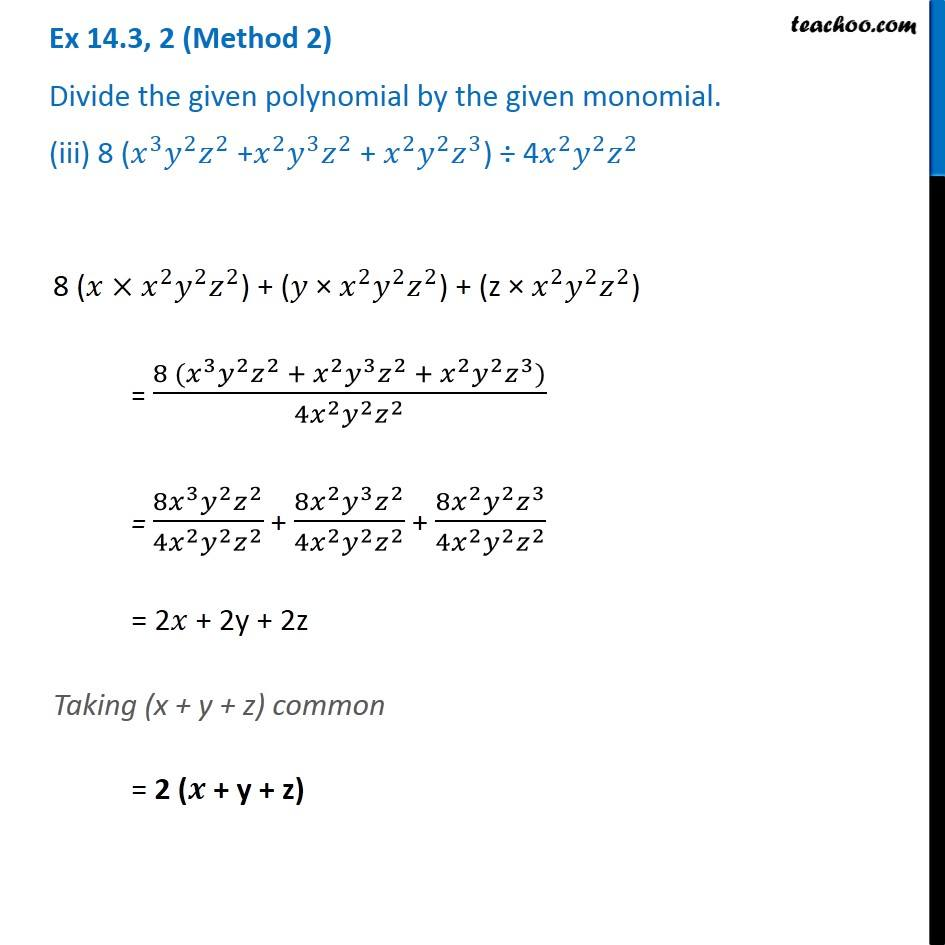Dividing polynomial by monomial

Chapter 12 Class 8 Factorisation
Concept wiseLearn in your speed, with individual attention - Teachoo Maths 1-on-1 Class

### Transcript

Ex 12.3, 2 (Method 1) Divide the given polynomial by the given monomial. (iii) 8 (𝑥^3 𝑦^2 𝑧^2 +𝑥^2 𝑦^3 𝑧^2 + 𝑥^2 𝑦^2 𝑧^3) ÷ 4𝑥^2 𝑦^2 𝑧^2 8 (𝑥^3 𝑦^2 𝑧^2 +𝑥^2 𝑦^3 𝑧^2 + 𝑥^2 𝑦^2 𝑧^3) = 8 (𝑥×𝑥^2 𝑦^2 𝑧^2) + (𝑦 × 𝑥^2 𝑦^2 𝑧^2) + (z × 𝑥^2 𝑦^2 𝑧^2) Taking 𝑥^2 𝑦^2 𝑧^2 common = 8𝑥^2 𝑦^2 𝑧^2 (𝑥 + y +z) Dividing (8 (𝑥^3 𝑦^2 𝑧^2 + 𝑥^2 𝑦^3 𝑧^2 + 𝑥^2 𝑦^2 𝑧^3))/(4𝑥^2 𝑦^2 𝑧^2 ) = (8〖 𝑥〗^2 𝑦^2 𝑧^2 (𝑥 + 𝑦 + 𝑧))/(4𝑥^2 𝑦^2 𝑧^2 ) = 8/4 × (𝑥^2 𝑦^2 𝑧^2)/(𝑥^2 𝑦^2 𝑧^2 ) × (𝑥 + y + z) = 2 × (𝑥 + y + z) = 2 (𝒙 + y + z) Ex 12.3, 2 (Method 2) Divide the given polynomial by the given monomial. (iii) 8 (𝑥^3 𝑦^2 𝑧^2 +𝑥^2 𝑦^3 𝑧^2 + 𝑥^2 𝑦^2 𝑧^3) ÷ 4𝑥^2 𝑦^2 𝑧^2 8 (𝑥×𝑥^2 𝑦^2 𝑧^2) + (𝑦 × 𝑥^2 𝑦^2 𝑧^2) + (z × 𝑥^2 𝑦^2 𝑧^2) = (8 (𝑥^3 𝑦^2 𝑧^2 + 𝑥^2 𝑦^3 𝑧^2 + 𝑥^2 𝑦^2 𝑧^3))/(4𝑥^2 𝑦^2 𝑧^2 ) = (8𝑥^3 𝑦^2 𝑧^2)/(4𝑥^2 𝑦^2 𝑧^2 ) + (8𝑥^2 𝑦^3 𝑧^2)/(4𝑥^2 𝑦^2 𝑧^2 ) + (8𝑥^2 𝑦^2 𝑧^3)/(4𝑥^2 𝑦^2 𝑧^2 ) = 2𝑥 + 2y + 2z Taking (x + y + z) common = 2 (𝒙 + y + z)